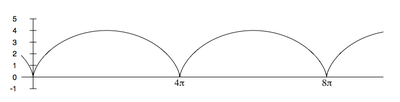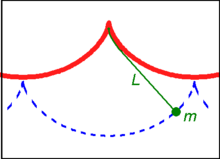# Cycloid

CycloidA cycloid generated by a rolling circle

A cycloid is the curve traced by a point on the rim of a circular wheel as the wheel rolls along a straight line. It is an example of a roulette, a curve generated by a curve rolling on another curve.

The cycloid is the solution to the brachistochrone problem (i.e. it is the curve of fastest descent under gravity) and the related tautochrone problem (i.e. the period of an object in descent without friction inside this curve does not depend on the object's starting position).

## History

The cycloid was first studied by Nicholas of Cusa and later by Mersenne. It was named by Galileo in 1599. In 1634 G.P. de Roberval showed that the area under a cycloid is three times the area of its generating circle. In 1658 Christopher Wren showed that the length of a cycloid are four times the diameter of its generating circle. The cycloid has been called "The Helen of Geometers" as it caused frequent quarrels among 17th century mathematicians.

## EquationsA cycloid generated by a circle of radius r = 2

The cycloid through the origin, generated by a circle of radius r, consists of the points (x, y), with$x = r(t - \sin t)\,$$y = r(1 - \cos t)\,$

where t is a real parameter, corresponding to the angle through which the rolling circle has rotated, measured in radians. For given t, the circle's centre lies at x = rt, y = r.

Solving for t and replacing, the Cartesian equation would be$x = r \cos^{-1} \left(1-\frac{y}{r}\right)-\sqrt{y(2r-y)}$

The first arch of the cycloid consists of points such that$0 \le t \le 2 \pi.\,$

The cycloid is differentiable everywhere except at the cusps where it hits the x-axis, with the derivative tending toward$\infty$ or$-\infty$ as one approaches a cusp. The map from t to (xy) is a differentiable curve or parametric curve of class C and the singularity where the derivative is 0 is an ordinary cusp.

The cycloid satisfies the differential equation:$\left(\frac{dy}{dx}\right)^2 = \frac{2r-y}{y}.$

## Area

One arch of a cycloid generated by a circle of radius r can be parameterized by$x = r(t - \sin t),\,$$y = r(1 - \cos t),\,$

with$0 \le t \le 2 \pi.\,$

Since$\frac{dx}{dt} = r(1- \cos t),$

we find the area under the arch to be\begin{align} A &= \int_{t=0}^{t=2 \pi} y \, dx = \int_{t=0}^{t=2 \pi} r^2(1-\cos t)^2 \, dt \\ &= \left. r^2 \left( \frac{3}{2}t-2\sin t + \frac{1}{2} \cos t \sin t\right) \right|_{t=0}^{t=2\pi} \\ &= 3 \pi r^2. \end{align}

## Arc length

The arc length S of one arch is given by\begin{align} S &= \int_{t=0}^{t=2 \pi} \left(\left(\frac{dy}{dt}\right)^2+\left(\frac{dx}{dt}\right)^2\right)^{1/2} \, dt \\ &= \int_{t=0}^{t=2 \pi} r \sqrt{2-2\cos(t)} \, dt \\ &= \int_{t=0}^{t=2 \pi} 2r \sin\left(\frac{t}{2}\right) \, dt \\ &= 8 r. \end{align}

## Cycloidal pendulumSchematic of a cycloidal pendulum.

If a simple pendulum is suspended from the cusp of an inverted cycloid, such that the "string" is constrained between the adjacent arcs of the cycloid, and the pendulum's length is equal to that of half the arc length of the cycloid (i.e. twice the diameter of the generating circle), the bob of the pendulum also traces a cycloid path. Such a cycloidal pendulum is isochronous, regardless of amplitude.

The 17th Century Dutch mathematician Christiaan Huygens discovered and proved these properties of the cycloid in the search to the design of more accurate pendulum clocks for use in navigation.

## Related curves

Several curves are related to the cycloid.

• Curtate cycloid: Here the point tracing out the curve is inside the circle, which rolls on a line.
• Prolate cycloid: Here the point tracing out the curve is outside the circle, which rolls on a line.
• Trochoid: refers to any of the cycloid, the curtate cycloid and the prolate cycloid.
• Hypocycloid: The point is on the edge of the circle, which rolls not on a line but on the inside of another circle.
• Epicycloid: The point is on the edge of the circle, which rolls not on a line but on the outside of another circle.
• Hypotrochoid: As hypocycloid but the point need not be on the edge of its circle.
• Epitrochoid: As epicycloid but the point need not be on the edge of its circle.

All these curves are roulettes with a circle rolled along a uniform curvature. The cycloid, epicycloids, and hypocycloids have the property that each is similar to its evolute. If q is the product of that curvature with the circle's radius, signed positive for epi- and negative for hypo-, then the curve:evolute similitude ratio is 1 + 2q.

The classic Spirograph toy traces out hypotrochoid and epitrochoid curves.

## Use in architectureCycloidal arches at the Kimbell Art Museum

The cycloidal arch was used by architect Louis Kahn in his design for the Kimbell Art Museum in Fort Worth, Texas. It was also used in the design of the Hopkins Center in Hanover, New Hampshire.

## Cited References

1. ^ Cajori, Florian (1999). A History of Mathematics. New York: Chelsea. p. 177. ISBN 978-0821821022.
2. ^ C. Huygens, "The Pendulum Clock or Geometrical Demonstrations Concerning the Motion of Pendula (sic) as Applied to Clocks," Translated by R. J. Blackwell, Iowa State University Press (Ames, Iowa, USA, 1986).

## Other References

• An application from physics: Ghatak, A. & Mahadevan, L. Crack street: the cycloidal wake of a cylinder tearing through a sheet. Physical Review Letters, 91, (2003). link.aps.org
• Edward Kasner & James Newman (1940) Mathematics and the Imagination, pp 196–200, Simon & Schuster.
• Wells D (1991). The Penguin Dictionary of Curious and Interesting Geometry. New York: Penguin Books. pp. 445–47. ISBN 0-14-011813-6.

Wikimedia Foundation. 2010.

### Look at other dictionaries:

• Cycloid — Cy cloid (s? kloid), n. [Cyclo + oid: cf. F. cyclo[ i]de.] (Geom.) A curve generated by a point in the plane of a circle when the circle is rolled along a straight line, keeping always in the same plane. [1913 Webster] Note: The common cycloid is …   The Collaborative International Dictionary of English

• Cycloid — Cy cloid, a. (Zo[ o]l.) Of or pertaining to the Cycloidei. [1913 Webster] {Cycloid scale} (Zo[ o]l.), a fish scale which is thin and shows concentric lines of growth, without serrations on the margin. [1913 Webster] …   The Collaborative International Dictionary of English

• Cycloid — Cy cloid, n. (Zo[ o]l.) One of the Cycloidei. [1913 Webster] …   The Collaborative International Dictionary of English

• cycloid — [sī′kloid΄] n. [Gr kykloeidēs, circular < kyklos (see WHEEL) + eidēs, OID] Geom. a curve traced by any point on a radius, or an extension of the radius, of a circle which rolls without slipping through one complete revolution along a straight… …   English World dictionary

• cycloid — cycloidal, adj. cycloidally, adv. /suy kloyd/, adj. 1. resembling a circle; circular. 2. (of the scale of a fish) smooth edged, more or less circular in form, and having concentric striations. 3. (of a fish) having such scales. 4. Psychiatry. of… …   Universalium

• cycloid — Suggesting cyclothymia; a term applied to a person who tends to have periods of marked swings of mood, but within normal limits. [cyclo + G. eidos, resembling] * * * cy·cloid sī .klȯid n a cycloid individual cycloid adj relating to, having, or… …   Medical dictionary

• cycloid — I. noun Etymology: French cycloïde, from Greek kykloeidēs circular, from kyklos Date: 1661 a curve that is generated by a point on the circumference of a circle as it rolls along a straight line • cycloidal adjective II. adjective Date: 1847 1.… …   New Collegiate Dictionary

• cycloid — Brachystochrone Bra*chys to*chrone, n. [Incorrect for brachistochrone, fr. Gr. bra chistos shortest (superl. of brachy s short) + ? time : cf. F. brachistochrone. ] (Math.) A curve, in which a body, starting from a given point, and descending… …   The Collaborative International Dictionary of English

• cycloid — 1. noun a) The locus of a point on the circumference of a circle that rolls without slipping on a fixed straight line. b) A fish having cycloid scales …   Wiktionary

• cycloid — /ˈsaɪklɔɪd/ (say suykloyd) adjective 1. resembling a circle; circular. 2. (of fishes scales) smooth edged, more or less circular in form, with concentric striations. 3. having such scales, as a fish. 4. Psychology (of a personality type)… …